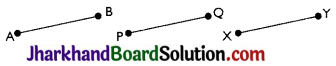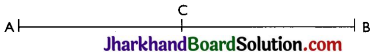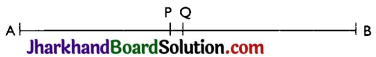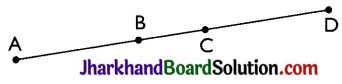# JAC Class 9 Maths Solutions Chapter 5 Introduction to Euclid’s Geometry Ex 5.1

Jharkhand Board JAC Class 9 Maths Solutions Chapter 5 Introduction to Euclid’s Geometry Ex 5.1 Textbook Exercise Questions and Answers.

## JAC Board Class 9th Maths Solutions Chapter 5 Introduction to Euclid’s Geometry Ex 5.1

Question 1.
Which of the following statements are true and which are false? Give reasons for your answers.
(i) Only one line can pass through a single point.
(ii) There are an infinite number of lines which pass through two distinct points.
(iii) A terminated line can be produced indefinitely on both the sides.
(iv) If two circles are equal, then their radii are equal.
(v) In Fig., if AB = PQ and PQ = XY, then AB = XY.(i) False. There can be infinite lines drawn passing through a single point.
(ii) False. Only one line can be drawn which passes through two distinct points.
(iii) True. A terminated line can be produced indefinitely on both the sides.
In geometry, a line can be extended in both the directions. A line means infinite long length.
(iv) True. If two circles are equal, then their radii are equal.
By superposition, we will find that the centre and circumference of the both circles coincide. Hence, their radii must be equal.
(v) True. By Euclid’s first axiom things which are equal to the same thing, are equal to one another.Question 2.
Give a definition for each of the following terms. Are there other terms that need to be defined first? What are they, and how might you define them?
(i) parallel lines
(ii) perpendicular lines
(iii) line segment
(v) square
Yes, other terms need to be defined first which are:
Plane: A plane is flat surface on which geometric figures are drawn.
Point: A point is a dot drawn on a plane surface and is dimensionless.
Line: A line is collection of points which can extend in both the directions and has only length not breadth.
(i) Parallel lines: When two or more lines never intersect each other in a plane and perpendicular distance between them is always constant then they are said to be parallel lines.
(ii) Perpendicular lines: When two lines intersect each other at right angle in a plane then they are said to be perpendicular to each other.
(iii) Line segment: A line segment is a part of a line with two end points and cannot be extended further.
(iv) Radius of a circle: The fixed distance between the centre and any point on the circumference of a circle is called the radius of the circle.
(v) Square: A square is a quadrilateral in which all the four sides are equal and each internal angle is a right angle.

Question 3.
Consider two ‘postulates’ given below:
(i) Given any two distinct points A and B, there exists a third point C which is in between A and B.
(ii) There exist at least three points that are not on the same line.
Do these postulates contain any undefined terms? Are these postulates consistent? Do they follow from Euclid’s postulates? Explain.
Undefined terms in the postulates:
⇒ Many points lie in a plane. But here it is not given about the position of the point C whether it lies on the line segment joining AB or not.
⇒ Also, there is no information about the plane whether the points are in same plane or not.
Yes, these postulates are consistent when we deal with these two situations:
(i) Point C is lying in between and on the line segment joining A and B.
(ii) Point C not lies on the line segment joining A and B.Page-86

Question 4.
If a point C lies between two points A and B such that AC = BC, then prove that AC = 1/2 AB. Explain by drawing the figure.Here, AC = BC
AC + AC = BC + AC
Also, BC + AC = AB (as it coincides with line segment AB)
∴ 2 AC = AB
⇒ AC = $$\frac{1}{2}$$ AB.

Question 5.
In Question 4, point C is called a mid-point of line segment AB. Prove that every line segment has one and only one mid-point.Let AB be the line segment and points P and Q be two different mid points of AB.
Now,
∴ P and Q are mid points of AB.
Therefore, AP = PB and also AQ = QB.
Also, PB + AP = AB (as it coincides with line segment AB)
Similarly, QB + AQ = AB.
Now, AP = PB
AP + AP = PB + AP
(If equals are added to equals, the wholes are equal.)
⇒ 2 AP = AB ……… (i)
Similarly,
2 AQ = AB ……. (ii)
From (i) and (ii)
2 AP = 2 AQ (Things which are equal to the same thing are equal to one another.)
⇒ AP = AQ
Thus, P and Q are the same points. This contradicts the fact that P and Q are two different mid points of AB. Thus, it is proved that every line segment has one and only one mid-point.

Question 6.
In Fig, if AC = BD, then prove that AB = CD.Given, AC = BD
From the figure,
AC = AB + BC
BD = BC + CD
⇒ AB + BC = BC + CD
According to Euclid’s axiom, when equals are subtracted from equals, remainders are also equal.
Subtracting BC from both sides,
AB + BC – BC = BC + CD – BC
AB = CDQuestion 7.
Why is Axiom 5, in the list of Euclid’s axioms, considered a ‘universal truth’? (Note that the question is not about the fifth postulate.)